How to Solve Polynomial Functions

Key Terms

o         Polynomial function

o         Subscript

o         Coefficient

o         Degree (of a polynomial)

o         Nonlinear function

o         Parabola

o         Factor

Objectives

o         Recognize a polynomial function

o         Know how many solutions a polynomial equation has

o         Learn how to factor quadratic expressions

o         Know how to use the quadratic formula

Polynomial Functions

Another type of function (which actually includes linear functions, as we will see) is the polynomial. A polynomial function is a function that is a sum of terms that each have the general form axn, where a and n are constants and x is a variable. Thus, a polynomial function p(x) has the following general form: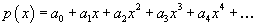Note that we use subscripts with the a factors (also referred to as coefficients) to make the representation of the function more uniform and lucid. When the function has a finite number of terms, the term with the largest value of n determines the degree of the polynomial: we say that the function is a polynomial of degree n (or an nth degree polynomial). Thus, a polynomial of degree n can be written as follows: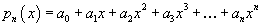Notice, then, that a linear function is a first-degree polynomial: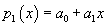f(x) = mx + b

Polynomials of a degree higher than one are nonlinear functions; that is, they do not plot graphically as a straight line. Instead, polynomials can have any particular shape depending on the number of terms and the coefficients of those terms. Finding the zeros of a polynomial function (recall that a zero of a function f(x) is the solution to the equation f(x) = 0) can be significantly more complex than finding the zeros of a linear function. For simplicity, we will focus primarily on second-degree polynomials, which are also called quadratic functions.

A quadratic function is a polynomial of degree two. Because it is common, we'll use the following notation when discussing quadratics:

f(x) = ax2 + bx + c

Let's take a look at the shape of a quadratic function on a graph. We'll just graph f(x) = x2.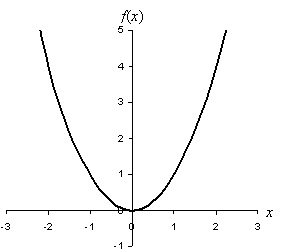The graph shows that the function is obviously nonlinear; the shape of a quadratic is actually a parabola. The quadratic function can be oriented either up (when a > 0, as in the above graph) or down (when a < 0), and it can be translated to any position in the plane (through variation of b and c). Note also that, depending on its location, the parabola can cross the x-axis in two places, in one place (as in the above graph), or nowhere at all. Generally, however, a quadratic equation has two solutions, which may or may not correspond to the same number. Furthermore, the solutions to a quadratic equation may be complex numbers. (In fact, it is generally the case that an equation consisting of a polynomial of degree n has n solutions.) There are two essential approaches to solving a quadratic equation: factoring and the quadratic formula.

A solution to any equation f(x) = 0 is the value or values of x for which f(x) is zero (that is, for which it crosses the x-axis). Let's look at the example quadratic function above:

f(x) = x2 = (x)(x)

What we have done here is factor the original expression. We can now see that this quadratic function has two zeros, both of which are at x = 0. But what if the function is more complicated? Let's first consider the following general quadratic expression. We can expand the expression by carefully applying the rule of distributivity.

f(x) = (px + q)(rx + s) = px(rx + s) + q(rx + s) = prx2 + pxs + qrx + qs

f(x) = prx2 + (ps + qr)x + qs

Note that this is fundamentally the same form as f(x) = ax2 + bx + c, but different names are used for the coefficients. The zeros of the function are the x values for which either factor is equal to zero-thus, we can see that there are generally two solutions to a quadratic. We can find these solutions by setting each factor equal to zero and solving for x. Of course, going from the factored form to the standard form is much more difficult than the reverse process, but in many cases the factored form can be found without too much difficulty. The process of factoring a quadratic function is usually a process of trial and error, but with practice, you can learn to spot how to factor some quadratics.

Practice Problem: Factor the expression x2 - 4.

Solution: This is our first problem involving factoring; start with what you know. We can write a quadratic expression as follows:

(px + q)(rx + s) = prx2 + (ps + qr)x + qs

More simply, we can assume p = r = 1 and write

(x + q)(x + s) = x2 + (s + q)x + qs

Now, we see that s must be equal to –q and that the product of s and q must be –4.

s + q = 0

s = –q

And,

sq = –4

–s2 = –4

s2 = 4

Now, s can be either 2 or –2, but it can only be one or the other. Let's just pick one: s = 2. Then, q = –2. Let's write the new expression and check our result:

(x + 2)(x – 2) = x(x – 2) + 2(x – 2) = x2 – 2x + 2x – 4 = x2 – 4

This result checks out.

Practice Problem: Factor the expression x2 + 3x + 2.

Solution: We can follow the same approach here as in the previous problem: our solution should have the following form:

(x + a)(x + b) = x2 + (a + b)x + ab = x2 + 3x + 2

So, we know that ab = 2 and a + b = 3. Here, trial and error is the best route. By inspection, a = 1 and b = 2 satisfies these equations.

ab = (1)(2) = 2

a + b = 1 + 2 = 3

b = 2

a = 1

The factored form is thus (x + 1)(x + 2). (Note that the zeros of the function are then x = –1 and x = –2.)

Practice Problem: Factor the expression x2 + 1.

Solution: Here we run into a slight problem. Let's take a look.

(x + q)(x + s) = x2 + (s + q)x + qs = x2 + 1

We see that q = –s, but also qs = 1.

qs = –s2 = 1

s2 = –1

s = ±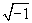Thus, we see that complex numbers can be involved in factors and zeros of quadratic functions. We can still handle this problem recalling what we already know about complex numbers.

s = i

q = –i

So, the factored expression is (x + i)(x – i). Let's expand this just to check.

(x + i)(xi) = x2 + ix – ix – i2 = x2 – (–1) = x2 + 1

The zeros of this expression are then i and –i. (As it turns out, complex solutions exist when the graph of the quadratic function does not cross the x-axis anywhere--try graphing the expression in this problem to see.)

A more general and direct way to find the zeros of a quadratic function (and, also, to find the factors) is through the quadratic formula. We will not derive the quadratic formula here, but suffice it to say you can derive it using algebra. Given a quadratic function ax2 + bx + c, the zeros of the function are at

x =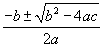Practice Problem: Find the solutions to the equation x2 – 4 = 0.

Solution: We can use the factoring approach, as we did in a previous practice problem, or we can use the quadratic formula with a = 1, b = 0, and c = –4. Let's try this latter approach to compare.

x =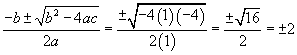The solutions to the equation are then x = 2 and x = –2. In some cases, the use of the quadratic equation is faster, even though factoring of the quadratic expression is still an option.